Courses

# Previous Year Questions (Part - 1) - Circles Class 10 Notes | EduRev

## Class 10 : Previous Year Questions (Part - 1) - Circles Class 10 Notes | EduRev

The document Previous Year Questions (Part - 1) - Circles Class 10 Notes | EduRev is a part of the Class 10 Course Mathematics (Maths) Class 10.
All you need of Class 10 at this link: Class 10

Short Answer Type Questions

Q.1. In fig., AB is a chord of length 8 cm of a circle of radius 5 cm. The tangents to the circle at A and B intersect at P. Find the length of AP.     [Delhi 2019, CBSE 2018 (C)]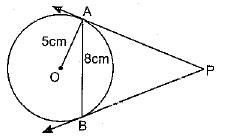Ans. Given: PA and PB are tangents to a circle.
Chord AB = 8 cm and radius OA = 5 cm
Construction: Join OP intersecting AB at M.
To find: Length AP
Solution: OP is perpendicular bisector of AB intersecting AB at M.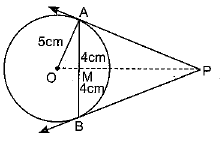In ΔOMA
OA2 = OM+AM2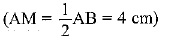(5)2 = OM2 + (4)2
25 = OM2 + 16
OM2 = 9 ⇒ OM - 3 cm
In ΔOAP, ∠OAP = 90°
(radius is perpendicular to tangent)
OP2 = OA2 + AP2
AP2 = OP2 - OA2         ...(i)
In ΔAMP, ∠AMP = 90°
AP2 = AM2 + PM2
= AM2 + (OP - OM)2 ...(ii)
Equating (i) and (ii), we get
OP2 - OA2 = AM2 + (OP - OM)2
⇒ OP2 - (5)2 = (4)2 + (OP - 3)2
⇒OP2 - 25 = 16 + OP2 + 9 - 6(OP)
⇒ - 6(OP) = - 50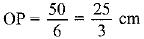∴  Eq (i) becomes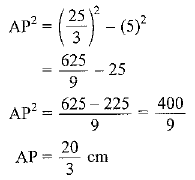Q.2.  Prove that the lengths of tangents drawn from an external point to a circle are equal.      [Delhi 2018]
Ans. Given: In circle, O is the centre. P is an external point and PA and PB are the tangents drawn.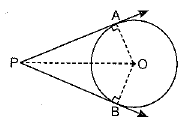To prove: PA = PB
Construction: Join OA, OB and OP.
Proof: Since, PA and PB are the tangents and OA and OB are the radii of a circle.
∴ OA ⊥ PA and OB ⊥ PB
[Tangent to a circle makes angle 90° with the radius at die point of contact]
⇒ ∠OAP = ∠OBP = 90°
Now, in ΔOAP and ΔOBP,
OA = OB (Radii)
OP = OP (Common)
∠OAP = ∠OBP (Each 90°)
ΔOAP ≌ ΔOBP
[By RHS congruence rule]
PA = PB [By CPCT]
Hence, proved.

Q.3. Two tangents TP and TQ are drawn to a circle with centre O from an external point T. Prove that ∠PTQ = 2∠OPQ.     [Delhi 2017]
Ans. Given: A circle with centre O.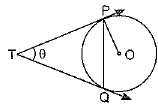An external point T from which TP and TQ are two tangents to the circle.
To prove: PTQ = 2OPQ
Proof: LetPTQ = θ
Now TP = TQ [Lengths of tangent segments from an external point to a circle are equal]
∴ ΔTPQ is an isosceles triangle.
⇒  TPQ = TQP [Angles opposite to equal sides of a triangle are equal]
In triangle TPQ
PTQ + TPQ + TQP = 180°
[Angle sum property of triangles]
⇒ θ + 2TPQ = 180°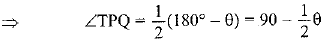Also, OPT = 90° [Angle between tangent and radius through the point of contact]
So, OPQ - ZOPT - TPQ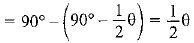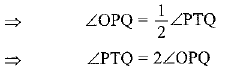Q.4. If the angle between two tangents drawn from an external point P to a circle of radius a and centre O, is 60°, then find the length of OP. [Delhi 2017]
Ans. Given: Radius of circle = a
∠QPR = 60°
In ΔPOQ and ΔPOR,
OQ = OR (radii), ∠Q = ∠R - (Each 90°), PQ = PR
(∵ PR and PQ are tangents from an external point P)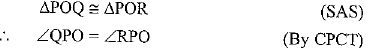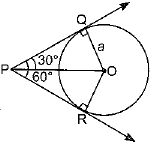Now, in right triangle OQP,
OQ/OP = sin 30º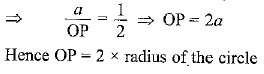Q.5. In the given fig., there are two concentric circles with centre O. PRT and PQS are tangents to the inner circle from a point P lying on the outer circle. If PR = 5 cm, find the length of PS     [Delhi 2017 (C)]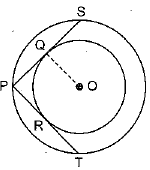Ans. PR and PQ are tangents to the external point P.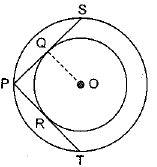∴ PR = PQ
∴ PQ = 5 c m (∵ PR = 5 cm)
PS = 2PQ (∵ OQ ⊥ PS)
= 2 x 5
= 10 cm

Q.6. In the given Fig., O is the centre of the circle, PQ is a chord and PT is tangent to the circle at P. If ∠POQ = 70°, find ∠TPQ.    [AI 2017 (C)]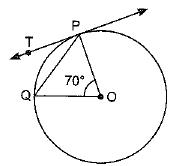Ans. Given: O is centre of the circle, PQ is chord and PT is tangent at P.
To find: ∠TPQ
Solution: In ΔOPQ
∠POQ + ∠OPQ + ∠OQP - 180°
⇒ 70° + ∠OPQ + ∠OPQ = 180°
(∵ OP = OQ, radii of the circle)
⇒ 70° + 2∠OPQ = 180°
⇒ 2∠OPQ = 110°
⇒ ∠OPQ = 55°
OP is perpendicular to the tangent at P.
∠OPT = 90°
⇒ ∠OPQ + ∠TPQ - 90°
⇒ 55° + ∠TPQ =90°
⇒ ∠TPQ = 90° - 55°
∠TPQ = 35°

Q.7. In the figure, AB and CD are common tangents to two circles of unequal radii. Prove that AB = CD.     [Delhi 2017]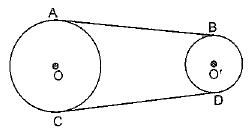Ans. Construction: Join AD and BC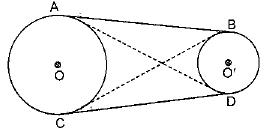Proof: We know that the lengths of tangents from an external point to a circle are equal.
So, if A is an external point for circle having centre O', then
AB = AD      ...(i)
If ‘C’ is an external point for circle with centre O', then
CB = CD ...(ii)
Also, B is an external point for circle with centre O,
∴  BC = AB ...(iii)
D is an external point for circle with centre O.
So DA = DC ...(iv)
So, from (i), (ii), (iii) and (iv), we get
AB = BC = CD
⇒ AB = CD Hence proved

Q.8. Prove that the tangents drawn at the end points of a chord of a circle make equal angles with the chord.     [AI 2017]
Sol.
Let AB be the chord of the circle with centre O.
Let PQ and RS respectively be the tangents at the end points A and B of the chord.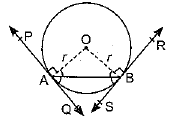Join OA and OB.
ΔOAB is an isosceles as OA = OB = r
[radii of the same circle]
∴ ∠OAB = ∠OBA     ...(i)
[Angles opposite to equal sides of a triangle are equal]
Now ∠OAP = ∠OBR      ... (ii)
[90° each angle between tangents and radius at the point of contact]
Adding equation (i) and equation (ii), we get
∠OAB + ∠OAP = ∠OBA + ∠OBR
⇒ ∠BAP = ∠ABR
Also, ∠OAQ = ∠OBS [90° each] ...(iii)
Subtracting equation (i) from equation (iii), wc got
∠OAQ - ∠OAB = ∠OBS - ∠OBA
∠BAQ = ∠ABS
Hence proved.

Q.9. In the given fig., PQ is a tangent from an external point P to a circle with centre O and OP cuts the circle at T and QOR is a diameter. If ∠POR = 130° and S is a point on the circle, find ∠1 + ∠2.    [Delhi 2017 (C)]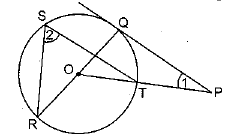Ans. To find: ∠1 + ∠2
OQ is perpendicular to the tangent PQ. (radius is perpendicular to the tangent at the point of contact).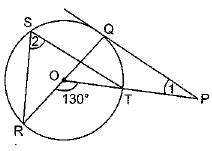In ΔOPQ
∠POR = ∠1 + ∠RQP
(exterior angle is equal to the .......... sum of interior opposite angles)
130° = ∠1 + 90°
∠1 = 130° - 90° = 40° ...(i)
∠ROT is the angle subtended by arc RT at centre and ∠RST is the angle subtended by arc RT at point S on circumference.
∴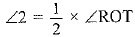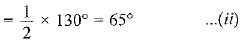Adding (i) and (ii), we get
∠1 + ∠2 = 40° + 65° = 105°

Q.10. In Fig., O is the centre of the circle and LN is a diameter. If PQ is a tangent to the circle at K and ∠KLN = 30°, find ∠PKL.   [AI 2017 (C)]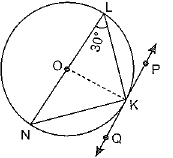Ans. Given: O is centre of circle and LN is the diameter, PQ is tangent at K, ∠KLN = 30°
To find: ∠PKL
Solution In ΔOKL, OL = OK (radii of a circle)
∴ ∠OLK = ∠OKL = 30°
(angle opposite to equal sides are equal)
OK is perpendicular to the tangent PQ at K.
∴ ∠OKP = 90°
⇒ ∠OKL + ∠LKP = 90°
⇒ 30° + ∠PKL = 90°
∴ ∠PKL = 60°

Q.11. In Fig. 3, the radius of in circle of ΔABC of area 84 cm2 is 4 cm and the lengths of the segments AP and BP into which side AB is divided by the point of contact P are 6 cm and 8 cm. Find the length of the sides AC and BC.    [AI 2017 (C)]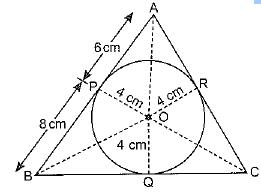Ans.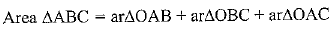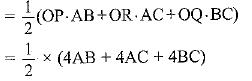84 = 2(AB + AC + BC)
42 = AB + AC + BC
⇒ AC + BC = 28 cm ...(i) [∵ AB = 14 cm]
Now, AP = AR ; BP = BQ
and CQ = CR = x (say)
(Length tangents from common exterior point)
So, AR = 6 cm, BQ = 8 cm, CQ = x
From eq (z) we have
AR + CR + CQ + BQ = 28
6 + x + x + 8 = 28
2x + 14 = 28
2x = 14
⇒ x = 7
∴ AC = AR + RC
= 6 + 7 = 13 cm
BC = BQ + QC
= 8 + 7 = 15 cm

Q.12. In Fig. 8.33, PA and PB are tangents to the circle from an external point P. CD is another tangent touching the circle at Q. If PA =12 cm, QC = QD = 3 cm, then find PC + PD.     [CBSE Delhi 2017]
Ans. PA = PC + CA = PC + CQ (∵ CA = CQ (tangents drawn from external point are equal)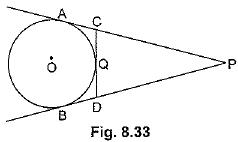⇒ 12 = PC + 3 ⇒ PC = 9 cm
∵ PA =PB ⇒ PA - AC = PB - BD ⇒ PC = PD
∴ PD = 9 cm
Hence, PC + PD = 18 cm

Q.13. The incircle of an isosceles triangle ABC, in which AB = AC, touches the sides BC, CA and AB at D, E and F respectively. Prove that BD = DC.  [CBSE (AI) 20 14]
OR
In Fig.8.32, if AB =AC, prove that BE = EC.       [CBSE (E) 2017]
[Note: D, E, F replace by F, D, E]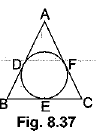Ans. Given, AB = AC (In Fig 8.37)
We have, BE + AF = AE + CE    ......(i)
AB, BC and CA are tangents to the circle at. F, D and E respectively.
∴  BF = BD, AE = AF and CE = CD       ....(ii)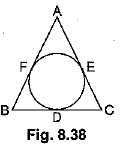From (i) and (ii)
BD + AE = AE + CD (∵ AF = AE)
⇒ BD = CD

Q.14. A quadrilateral ABCD is drawn to circumscribe a circle (Fig. 8.55). Prove that AB + CD = AD + BC.      [NCERT, CBSE (AI) 2016]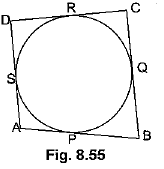OR
A circle touches all the four sides o f a quadrilateral ABCD. Prove that AB + CD = BC + DA     [CBSE (AI) 2017, Delhi 2017 (C)]
Ans. Since lengths of two tangents drawn from an external point of circle are equal,
Therefore, AP = AS, BP = BQ, DR = DS and CR = CQ
(where P, Q, R and S are the points of contact)
Adding all these, we have
(AP + BP) + (CR + RD) = (BQ + CQ) + (DS + AS)
⇒ AB + CD = BC + DA

Offer running on EduRev: Apply code STAYHOME200 to get INR 200 off on our premium plan EduRev Infinity!

## Mathematics (Maths) Class 10

51 videos|346 docs|103 tests

,

,

,

,

,

,

,

,

,

,

,

,

,

,

,

,

,

,

,

,

,

;